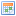# ConVars

 Lua: ConVarsDescription: An introduction in ConVars.Original Author: Assault_TrooperCreated: October 24th 2010

## Explaining ConVars

### CreateClientConVar

This is propably the most common convar function used, it will create a client-sided convar and you can change it from your own console. And as the name tells you, it's client-sided.

```
CreateClientConVar( "test_enabled", 0, true, false )
```

or if you want to have a variable accorded to the convar, you could do it like this.

```
local TestConvar = CreateClientConVar( "test_enabled", 0, true, false )
```

### CreateConVar

If you would want to have a convar that can only be changed via the rcon, you would do this. This is server-sided. This will only appear and work on the servers rcon.

```
CreateConVar( "test_enabled", 0, FCVAR_SERVER_CAN_EXECUTE )
```

If you would want to have it work on clients console, and rcon, you would do this.

```
CreateConVar( "test_enabled", 0, { FCVAR_SERVER_CAN_EXECUTE, FCVAR_CLIENTCMD_CAN_EXECUTE } )
```

### GetConVar

And sometimes, you will want to read the convar, and this is how you'll do it.

```
GetConVar( "test_enabled" )
```

And now, if we would want to read the convars value, we would do this.

```
local Value = GetConVar( "test_enabled" ):GetInt()
```

### ConVarExists

Another useful function is, ConVarExists. With it, we can see if the convar exists, and then do some operations on it. More info on the ConVarExists page.

```
if ConVarExists( "test_enabled" ) then
print( "Convar exists!" )
else
print( "Convar doesn't exist!" )
end
```

This might be useful when you want to retrieve a value from a convar, because if the convar doesn't exist, it will error.

```
if ConVarExists( "test_enabled" ) then
Value = GetConVar( "test_enabled" ):GetInt()
end
```